# Mean State

Period Mean (original grids) [Watt m-2]
Model Period Mean (intersection) [Watt m-2]
Model Period Mean (complement) [Watt m-2]
Benchmark Period Mean (intersection) [Watt m-2]
Benchmark Period Mean (complement) [Watt m-2]
Bias [Watt m-2]
RMSE [Watt m-2]
Phase Shift [months]
Bias Score 
RMSE Score 
Seasonal Cycle Score 
Spatial Distribution Score 
Interannual Variability Score 
Overall Score 
Benchmark [-] -56.8
CLM4 [-] -51.3 -51.0 -57.0 -52.3 6.43 15.0 2.14 0.512 0.456 0.672 0.982 0.573 0.608
CLM4.5 [-] -55.3 -55.3 -57.0 -52.3 2.36 15.8 2.17 0.494 0.455 0.668 0.977 0.574 0.604
CLM5 [-] -56.5 -56.4 -57.0 -52.3 1.23 14.0 2.12 0.586 0.458 0.676 0.992 0.553 0.621
Period Mean (original grids) [Watt m-2]
Model Period Mean (intersection) [Watt m-2]
Model Period Mean (complement) [Watt m-2]
Benchmark Period Mean (intersection) [Watt m-2]
Benchmark Period Mean (complement) [Watt m-2]
Bias [Watt m-2]
RMSE [Watt m-2]
Phase Shift [months]
Bias Score 
RMSE Score 
Seasonal Cycle Score 
Spatial Distribution Score 
Interannual Variability Score 
Overall Score 
Benchmark [-] -87.0
CLM4 [-] -83.4 -84.3 -87.4 -58.4 3.55 18.6 0.234 0.801 0.583 0.978 0.990 0.570 0.751
CLM4.5 [-] -88.6 -89.3 -87.4 -58.4 -1.45 19.5 0.294 0.752 0.588 0.974 0.875 0.567 0.724
CLM5 [-] -90.0 -90.7 -87.4 -58.4 -2.82 19.4 0.297 0.763 0.582 0.972 0.913 0.530 0.724
Period Mean (original grids) [Watt m-2]
Model Period Mean (intersection) [Watt m-2]
Model Period Mean (complement) [Watt m-2]
Benchmark Period Mean (intersection) [Watt m-2]
Benchmark Period Mean (complement) [Watt m-2]
Bias [Watt m-2]
RMSE [Watt m-2]
Phase Shift [months]
Bias Score 
RMSE Score 
Seasonal Cycle Score 
Spatial Distribution Score 
Interannual Variability Score 
Overall Score 
Benchmark [-] -81.4
CLM4 [-] -77.1 -77.3 -82.3 -62.6 6.74 19.5 1.50 0.682 0.488 0.784 0.996 0.528 0.661
CLM4.5 [-] -82.8 -83.0 -82.3 -62.6 1.65 19.8 1.46 0.684 0.482 0.790 0.989 0.545 0.662
CLM5 [-] -80.9 -81.1 -82.3 -62.6 3.32 20.0 1.18 0.704 0.474 0.845 0.993 0.501 0.665
Period Mean (original grids) [Watt m-2]
Model Period Mean (intersection) [Watt m-2]
Model Period Mean (complement) [Watt m-2]
Benchmark Period Mean (intersection) [Watt m-2]
Benchmark Period Mean (complement) [Watt m-2]
Bias [Watt m-2]
RMSE [Watt m-2]
Phase Shift [months]
Bias Score 
RMSE Score 
Seasonal Cycle Score 
Spatial Distribution Score 
Interannual Variability Score 
Overall Score 
Benchmark [-] -37.2
CLM4 [-] -52.3 -52.3 -36.9 -41.0 -15.6 20.2 1.04 0.391 0.475 0.880 0.807 0.571 0.600
CLM4.5 [-] -54.1 -54.1 -36.9 -41.0 -17.0 21.0 1.07 0.356 0.485 0.876 0.933 0.544 0.613
CLM5 [-] -60.3 -60.4 -36.9 -41.0 -23.3 26.1 1.03 0.242 0.494 0.878 0.828 0.503 0.573
Period Mean (original grids) [Watt m-2]
Model Period Mean (intersection) [Watt m-2]
Model Period Mean (complement) [Watt m-2]
Benchmark Period Mean (intersection) [Watt m-2]
Benchmark Period Mean (complement) [Watt m-2]
Bias [Watt m-2]
RMSE [Watt m-2]
Phase Shift [months]
Bias Score 
RMSE Score 
Seasonal Cycle Score 
Spatial Distribution Score 
Interannual Variability Score 
Overall Score 
Benchmark [-] -44.6
CLM4 [-] -23.1 -23.1 -43.2 -49.2 20.4 21.9 3.04 0.0675 0.361 0.491 0.861 0.558 0.450
CLM4.5 [-] -24.7 -24.7 -43.2 -49.2 19.3 21.0 3.04 0.0752 0.365 0.488 0.881 0.546 0.453
CLM5 [-] -29.4 -29.3 -43.2 -49.2 14.9 17.2 2.67 0.114 0.374 0.554 0.877 0.524 0.469
Period Mean (original grids) [Watt m-2]
Model Period Mean (intersection) [Watt m-2]
Model Period Mean (complement) [Watt m-2]
Benchmark Period Mean (intersection) [Watt m-2]
Benchmark Period Mean (complement) [Watt m-2]
Bias [Watt m-2]
RMSE [Watt m-2]
Phase Shift [months]
Bias Score 
RMSE Score 
Seasonal Cycle Score 
Spatial Distribution Score 
Interannual Variability Score 
Overall Score 
Benchmark [-] -42.1
CLM4 [-] -59.0 -58.9 -41.9 -51.7 -17.0 20.4 0.853 0.349 0.507 0.906 0.801 0.558 0.605
CLM4.5 [-] -59.4 -59.4 -41.9 -51.7 -17.5 20.9 0.897 0.337 0.497 0.900 0.895 0.576 0.617
CLM5 [-] -65.8 -65.8 -41.9 -51.7 -23.9 27.0 1.08 0.229 0.461 0.874 0.755 0.540 0.553
Period Mean (original grids) [Watt m-2]
Model Period Mean (intersection) [Watt m-2]
Model Period Mean (complement) [Watt m-2]
Benchmark Period Mean (intersection) [Watt m-2]
Benchmark Period Mean (complement) [Watt m-2]
Bias [Watt m-2]
RMSE [Watt m-2]
Phase Shift [months]
Bias Score 
RMSE Score 
Seasonal Cycle Score 
Spatial Distribution Score 
Interannual Variability Score 
Overall Score 
Benchmark [-] -67.1
CLM4 [-] -77.1 -76.9 -67.3 -59.4 -8.57 19.3 1.13 0.575 0.483 0.848 0.967 0.564 0.653
CLM4.5 [-] -77.8 -77.7 -67.3 -59.4 -9.28 19.4 1.14 0.568 0.485 0.845 0.968 0.563 0.652
CLM5 [-] -82.2 -82.1 -67.3 -59.4 -13.7 22.2 1.16 0.483 0.466 0.837 0.937 0.542 0.622
Period Mean (original grids) [Watt m-2]
Model Period Mean (intersection) [Watt m-2]
Model Period Mean (complement) [Watt m-2]
Benchmark Period Mean (intersection) [Watt m-2]
Benchmark Period Mean (complement) [Watt m-2]
Bias [Watt m-2]
RMSE [Watt m-2]
Phase Shift [months]
Bias Score 
RMSE Score 
Seasonal Cycle Score 
Spatial Distribution Score 
Interannual Variability Score 
Overall Score 
Benchmark [-] -71.2
CLM4 [-] -66.5 -66.6 -72.6 -53.4 10.3 21.4 1.06 0.594 0.521 0.873 0.982 0.543 0.672
CLM4.5 [-] -70.7 -70.8 -72.6 -53.4 6.52 20.6 1.05 0.635 0.522 0.872 0.981 0.539 0.679
CLM5 [-] -72.7 -72.7 -72.6 -53.4 4.54 20.1 1.01 0.661 0.517 0.868 0.986 0.494 0.674
Period Mean (original grids) [Watt m-2]
Model Period Mean (intersection) [Watt m-2]
Model Period Mean (complement) [Watt m-2]
Benchmark Period Mean (intersection) [Watt m-2]
Benchmark Period Mean (complement) [Watt m-2]
Bias [Watt m-2]
RMSE [Watt m-2]
Phase Shift [months]
Bias Score 
RMSE Score 
Seasonal Cycle Score 
Spatial Distribution Score 
Interannual Variability Score 
Overall Score 
Benchmark [-] -31.1
CLM4 [-] -50.4 -50.5 -31.1 -32.1 -19.4 22.2 0.796 0.296 0.515 0.932 0.977 0.584 0.636
CLM4.5 [-] -50.6 -50.7 -31.1 -32.1 -19.5 22.2 0.817 0.295 0.510 0.928 0.997 0.579 0.636
CLM5 [-] -58.4 -58.5 -31.1 -32.1 -27.2 29.3 0.789 0.183 0.499 0.932 0.958 0.528 0.600
Period Mean (original grids) [Watt m-2]
Model Period Mean (intersection) [Watt m-2]
Model Period Mean (complement) [Watt m-2]
Benchmark Period Mean (intersection) [Watt m-2]
Benchmark Period Mean (complement) [Watt m-2]
Bias [Watt m-2]
RMSE [Watt m-2]
Phase Shift [months]
Bias Score 
RMSE Score 
Seasonal Cycle Score 
Spatial Distribution Score 
Interannual Variability Score 
Overall Score 
Benchmark [-] -96.8
CLM4 [-] -94.4 -94.5 -99.4 -63.9 8.01 18.9 0.574 0.605 0.523 0.914 0.892 0.541 0.666
CLM4.5 [-] -94.7 -94.7 -99.4 -63.9 7.72 18.8 0.566 0.614 0.522 0.917 0.882 0.549 0.667
CLM5 [-] -96.7 -96.7 -99.4 -63.9 5.96 19.5 0.614 0.606 0.507 0.908 0.905 0.507 0.657
Period Mean (original grids) [Watt m-2]
Model Period Mean (intersection) [Watt m-2]
Model Period Mean (complement) [Watt m-2]
Benchmark Period Mean (intersection) [Watt m-2]
Benchmark Period Mean (complement) [Watt m-2]
Bias [Watt m-2]
RMSE [Watt m-2]
Phase Shift [months]
Bias Score 
RMSE Score 
Seasonal Cycle Score 
Spatial Distribution Score 
Interannual Variability Score 
Overall Score 
Benchmark [-] -55.6
CLM4 [-] -66.3 -66.3 -55.1 -60.8 -10.9 16.9 1.03 0.400 0.462 0.873 0.701 0.601 0.583
CLM4.5 [-] -65.7 -65.7 -55.1 -60.8 -10.3 16.4 1.08 0.408 0.470 0.860 0.640 0.585 0.572
CLM5 [-] -70.2 -70.3 -55.1 -60.8 -14.6 19.5 1.21 0.338 0.457 0.835 0.547 0.534 0.528
Period Mean (original grids) [Watt m-2]
Model Period Mean (intersection) [Watt m-2]
Model Period Mean (complement) [Watt m-2]
Benchmark Period Mean (intersection) [Watt m-2]
Benchmark Period Mean (complement) [Watt m-2]
Bias [Watt m-2]
RMSE [Watt m-2]
Phase Shift [months]
Bias Score 
RMSE Score 
Seasonal Cycle Score 
Spatial Distribution Score 
Interannual Variability Score 
Overall Score 
Benchmark [-] -52.6
CLM4 [-] -66.8 -66.8 -65.2 -46.5 -0.108 20.6 1.25 0.485 0.481 0.825 0.925 0.530 0.621
CLM4.5 [-] -68.3 -68.3 -65.2 -46.5 -1.39 20.4 1.21 0.486 0.482 0.834 0.927 0.531 0.624
CLM5 [-] -71.7 -71.7 -65.2 -46.5 -4.65 22.3 1.23 0.451 0.472 0.829 0.909 0.501 0.606
Period Mean (original grids) [Watt m-2]
Model Period Mean (intersection) [Watt m-2]
Model Period Mean (complement) [Watt m-2]
Benchmark Period Mean (intersection) [Watt m-2]
Benchmark Period Mean (complement) [Watt m-2]
Bias [Watt m-2]
RMSE [Watt m-2]
Phase Shift [months]
Bias Score 
RMSE Score 
Seasonal Cycle Score 
Spatial Distribution Score 
Interannual Variability Score 
Overall Score 
Benchmark [-] -121.
CLM4 [-] -102. -102. -122. -81.3 21.4 32.1 0.870 0.488 0.485 0.888 0.802 0.442 0.598
CLM4.5 [-] -102. -102. -122. -81.3 21.5 32.1 0.879 0.484 0.483 0.887 0.814 0.441 0.599
CLM5 [-] -103. -103. -122. -81.3 20.0 32.1 0.847 0.501 0.471 0.887 0.803 0.429 0.593
Period Mean (original grids) [Watt m-2]
Model Period Mean (intersection) [Watt m-2]
Model Period Mean (complement) [Watt m-2]
Benchmark Period Mean (intersection) [Watt m-2]
Benchmark Period Mean (complement) [Watt m-2]
Bias [Watt m-2]
RMSE [Watt m-2]
Phase Shift [months]
Bias Score 
RMSE Score 
Seasonal Cycle Score 
Spatial Distribution Score 
Interannual Variability Score 
Overall Score 
Benchmark [-] -34.3
CLM4 [-] -53.4 -53.4 -34.2 -36.5 -18.9 21.8 0.814 0.296 0.495 0.914 0.923 0.535 0.610
CLM4.5 [-] -53.7 -53.7 -34.2 -36.5 -19.1 21.9 0.738 0.291 0.499 0.921 0.934 0.531 0.612
CLM5 [-] -60.2 -60.2 -34.2 -36.5 -25.5 27.5 0.657 0.188 0.502 0.928 0.958 0.504 0.597
Period Mean (original grids) [Watt m-2]
Model Period Mean (intersection) [Watt m-2]
Model Period Mean (complement) [Watt m-2]
Benchmark Period Mean (intersection) [Watt m-2]
Benchmark Period Mean (complement) [Watt m-2]
Bias [Watt m-2]
RMSE [Watt m-2]
Phase Shift [months]
Bias Score 
RMSE Score 
Seasonal Cycle Score 
Spatial Distribution Score 
Interannual Variability Score 
Overall Score 
Benchmark [-] -70.7
CLM4 [-] -72.4 -72.4 -73.4 -57.5 6.50 17.3 1.29 0.531 0.472 0.795 0.982 0.592 0.641
CLM4.5 [-] -70.7 -70.7 -73.4 -57.5 8.61 18.4 1.39 0.503 0.467 0.782 0.940 0.593 0.625
CLM5 [-] -73.0 -73.0 -73.4 -57.5 6.44 17.5 1.72 0.533 0.463 0.721 0.957 0.538 0.613
Period Mean (original grids) [Watt m-2]
Model Period Mean (intersection) [Watt m-2]
Model Period Mean (complement) [Watt m-2]
Benchmark Period Mean (intersection) [Watt m-2]
Benchmark Period Mean (complement) [Watt m-2]
Bias [Watt m-2]
RMSE [Watt m-2]
Phase Shift [months]
Bias Score 
RMSE Score 
Seasonal Cycle Score 
Spatial Distribution Score 
Interannual Variability Score 
Overall Score 
Benchmark [-] -53.2
CLM4 [-] -59.1 -58.9 -52.9 -58.5 -5.19 18.5 4.03 0.537 0.288 0.309 0.896 0.691 0.502
CLM4.5 [-] -60.4 -60.1 -52.9 -58.5 -6.31 18.5 3.84 0.510 0.299 0.350 0.886 0.702 0.508
CLM5 [-] -66.4 -66.2 -52.9 -58.5 -12.3 21.4 4.41 0.367 0.283 0.259 0.923 0.641 0.459
Period Mean (original grids) [Watt m-2]
Model Period Mean (intersection) [Watt m-2]
Model Period Mean (complement) [Watt m-2]
Benchmark Period Mean (intersection) [Watt m-2]
Benchmark Period Mean (complement) [Watt m-2]
Bias [Watt m-2]
RMSE [Watt m-2]
Phase Shift [months]
Bias Score 
RMSE Score 
Seasonal Cycle Score 
Spatial Distribution Score 
Interannual Variability Score 
Overall Score 
Benchmark [-] -42.5
CLM4 [-] -60.5 -60.5 -42.4 -45.4 -18.2 21.6 0.807 0.339 0.512 0.893 0.917 0.537 0.618
CLM4.5 [-] -61.3 -61.4 -42.4 -45.4 -19.0 22.3 0.798 0.323 0.500 0.899 0.905 0.540 0.611
CLM5 [-] -67.9 -67.9 -42.4 -45.4 -25.4 28.5 1.02 0.223 0.461 0.860 0.707 0.526 0.540
Period Mean (original grids) [Watt m-2]
Model Period Mean (intersection) [Watt m-2]
Model Period Mean (complement) [Watt m-2]
Benchmark Period Mean (intersection) [Watt m-2]
Benchmark Period Mean (complement) [Watt m-2]
Bias [Watt m-2]
RMSE [Watt m-2]
Phase Shift [months]
Bias Score 
RMSE Score 
Seasonal Cycle Score 
Spatial Distribution Score 
Interannual Variability Score 
Overall Score 
Benchmark [-] -80.3
CLM4 [-] -85.3 -85.0 -80.8 -54.9 -3.87 19.1 0.429 0.699 0.547 0.950 0.762 0.554 0.676
CLM4.5 [-] -86.0 -85.7 -80.8 -54.9 -4.50 18.5 0.380 0.695 0.562 0.954 0.762 0.558 0.682
CLM5 [-] -90.6 -90.4 -80.8 -54.9 -9.08 21.9 0.524 0.622 0.525 0.929 0.712 0.518 0.638
Period Mean (original grids) [Watt m-2]
Model Period Mean (intersection) [Watt m-2]
Model Period Mean (complement) [Watt m-2]
Benchmark Period Mean (intersection) [Watt m-2]
Benchmark Period Mean (complement) [Watt m-2]
Bias [Watt m-2]
RMSE [Watt m-2]
Phase Shift [months]
Bias Score 
RMSE Score 
Seasonal Cycle Score 
Spatial Distribution Score 
Interannual Variability Score 
Overall Score 
Benchmark [-] -43.7
CLM4 [-] -27.6 -27.7 -43.6 -47.7 15.7 19.3 1.46 0.265 0.442 0.799 0.685 0.561 0.532
CLM4.5 [-] -30.5 -30.4 -43.6 -47.7 13.2 17.7 1.36 0.300 0.442 0.816 0.666 0.569 0.539
CLM5 [-] -31.4 -31.4 -43.6 -47.7 12.2 16.3 1.56 0.335 0.449 0.783 0.750 0.557 0.554
Period Mean (original grids) [Watt m-2]
Model Period Mean (intersection) [Watt m-2]
Model Period Mean (complement) [Watt m-2]
Benchmark Period Mean (intersection) [Watt m-2]
Benchmark Period Mean (complement) [Watt m-2]
Bias [Watt m-2]
RMSE [Watt m-2]
Phase Shift [months]
Bias Score 
RMSE Score 
Seasonal Cycle Score 
Spatial Distribution Score 
Interannual Variability Score 
Overall Score 
Benchmark [-] -64.5
CLM4 [-] -58.4 -58.8 -64.8 -56.3 6.71 18.0 1.40 0.491 0.481 0.800 0.595 0.582 0.572
CLM4.5 [-] -61.5 -62.0 -64.8 -56.3 3.80 16.0 1.35 0.566 0.480 0.811 0.658 0.593 0.598
CLM5 [-] -63.0 -63.4 -64.8 -56.3 2.33 15.8 1.19 0.602 0.473 0.837 0.663 0.537 0.597
Period Mean (original grids) [Watt m-2]
Model Period Mean (intersection) [Watt m-2]
Model Period Mean (complement) [Watt m-2]
Benchmark Period Mean (intersection) [Watt m-2]
Benchmark Period Mean (complement) [Watt m-2]
Bias [Watt m-2]
RMSE [Watt m-2]
Phase Shift [months]
Bias Score 
RMSE Score 
Seasonal Cycle Score 
Spatial Distribution Score 
Interannual Variability Score 
Overall Score 
Benchmark [-] -80.0
CLM4 [-] -90.1 -89.8 -83.0 -54.0 -4.70 23.4 0.951 0.438 0.453 0.876 0.656 0.513 0.565
CLM4.5 [-] -92.3 -92.0 -83.0 -54.0 -6.68 23.7 0.979 0.427 0.453 0.874 0.677 0.517 0.567
CLM5 [-] -94.1 -93.9 -83.0 -54.0 -8.56 24.9 1.07 0.414 0.455 0.854 0.617 0.488 0.547

# Temporally integrated period mean

BENCHMARK MEAN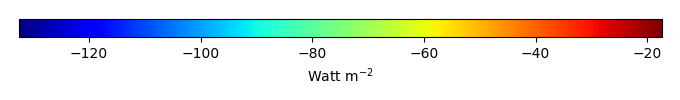MODEL MEANBIAS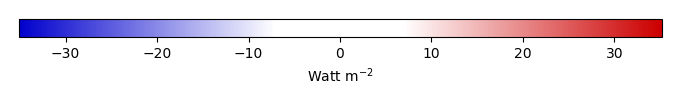BIAS SCORERMSE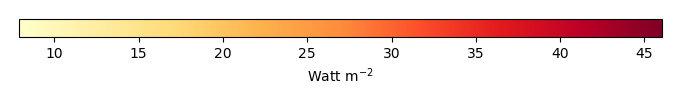RMSE SCOREBENCHMARK INTERANNUAL VARIABILITY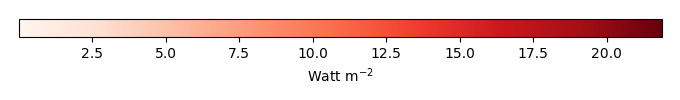MODEL INTERANNUAL VARIABILITYINTERANNUAL VARIABILITY SCOREBENCHMARK MAX MONTHMODEL MAX MONTHDIFFERENCE IN MAX MONTH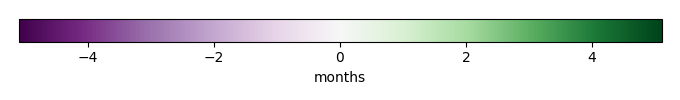SEASONAL CYCLE SCORESPATIAL TAYLOR DIAGRAMMODEL COLORS# Spatially integrated regional mean

MODEL COLORSREGIONAL MEANANNUAL CYCLEMONTHLY ANOMALYANNUAL CYCLE# All Models

BenchmarkCLM4CLM4.5CLM5# Data Information

creation_date: Tue Jul 1 08:25:00 PDT 2014

source_file: This product is generated from monthly 1 degree GEWEX SRB Radiation observations